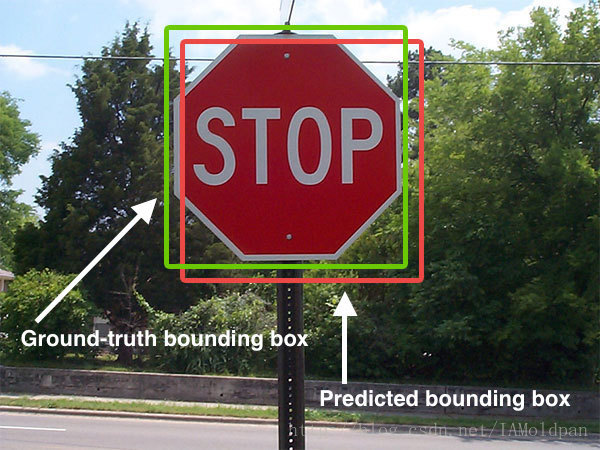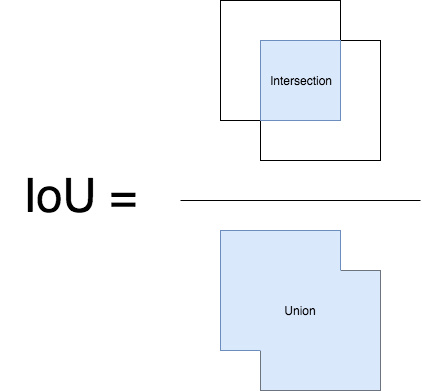## IoU (Intersection over Union)

IoU 是一个简单的测量标准，只要是在输出中得出一个预测范围(bounding boxex)的任务都可以用 IoU 来进行测量。为了可以使 IoU 用于测量任意大小形状的物体检测，我们需要：

1. ground-truth bounding boxes（人为在训练集图像中标出要检测物体的大概范围）；
2. 我们的算法得出的结果范围。$$IoU=\frac {Area\ of\ Overlap}{Area\ of\ Union}$$

python 实现

## mAP (Mean Average Precision)

1. 对于某个类别C，在某一张图片上首先计算C在一张图片上的 $$Precision=\frac {在一张图片上类别C识别正确的个数（也就是IoU>0.5）}{一张图片上类别C的总个数}$$

$$Precision_C\ =\ \frac {N(TruePositives)_C}{N(TotalObjects)_C}$$

1. 依然对于某个类别C，可能在多张图片上有该类别，下面计算类别C的AP指数： $$AP\ =\ \frac {每张图片上的Precision求和}{含有类别C的图片数目}$$

$$AveragePrecision_C\ =\ \frac {\sum Precision_C}{N(TotalImages)_C}$$

1. 对于整个数据集，存在多个类别C1、C2、C3： $$mAP\ =\ \frac {上一步计算的所有类别的AP和}{总的类别数目相当于所有类别的AP的平均值}$$

$$MeanAveragePrecision\ =\ \frac {\sum AveragePrecision_C}{N(Classes)}$$